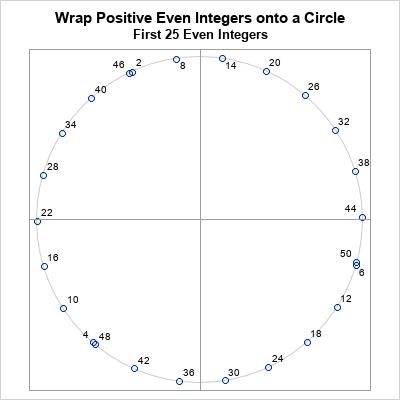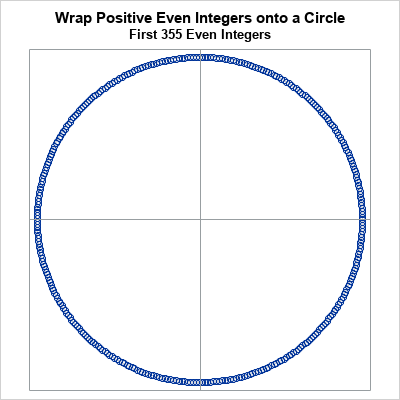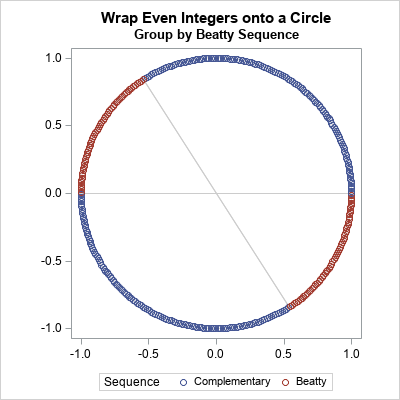On this Pi Day, let's explore the "πth roots of unity." (Pi Day is celebrated in the US on 3/14 to celebrate π ≈ 3.14159....) It's okay if you've never heard of the πth roots of unity. This article starts by reviewing the better-known nth roots of unity. It then shows all the math you'll need to understand the πth roots of unity and how to visualize them!

### The nth roots of unity

Recall that the nth root of unity for a positive integer, n, is a complex number, z, such that the nth power of z equals 1. In symbols, z satisfies the equation zn = 1.

For any positive integer, n, there are n roots of unity. The nth roots of unity are the complex numbers
$\exp(2\pi i k /n) = \cos(2 \pi k/n) + i \sin(2 \pi k/n)$
for k=0, 1, 2, ..., n. Geometrically, these points are the equally spaced points on the unit circle. You get them by tracing the image of the point 1 + 0*i as you rotate the circle by 2π/n radians. Some simple examples are:

• n=2. The square roots of unity are the numbers 1 and -1. Geometrically, these points are represented in the Argand plane by the ordered pairs (1, 0) and (-1, 0). They are the orbit of the point (1,0) under rotations by π radians.
• n=3. The cube roots of unity are the numbers 1, -1/2 + i sqrt(3)/2, and -1/2 - i sqrt(3)/2. These points are the orbit of the point (1,0) under rotations by 2π/3 radians.
• n=4. The 4th roots of unity are the numbers 1, i, -1, and -i. The coordinates of these points on the unit circle are (1,0), (0, 1), (-1, 0), and (0, -1). These points are the orbit of the point (1,0) under rotations by π/2 radians.

### The πth roots of unity

So, what is a πth root of unity? It is complex number, z, such that zπ = 1. Geometrically, it is one of the points on the orbit of the point (1,0) by rotations of 2*pi/pi = 2 radians. These complex numbers have the formula $z = \exp(2 i n)$ for any integer, n. You can verify that these numbers are the πth roots of unity by showing that $z^\pi = 1$, as follows:
$\exp(2 i n)^\pi = \exp(2\pi i n) = \cos(2 \pi n) + i \sin(2 \pi n) = 1$ for all integers n.

Notice that the "circle map" x → exp(i x) sends the even integers to the πth roots of unity. Thus, you can also refer to the points as the image of the even integers under the mapping.

Geometrically, the πth roots are generated by rotating the unit circle by 2 radians or by -2 radians. For simplicity, the subsequent sections visualize the πth roots for only the positive integers, which correspond to counterclockwise rotations by 2 radians.

### Visualize the πth roots of unity

Let's generate some positive integers and plot the complex points exp(2 i n) in the Argand plane. To get started, let's plot only the images of the first 25 even integers:

%let maxN = 355; data Roots; do n = 1 to &maxN; s = 2*n; xn = cos(s); /* image of even integers under mapping to unit circle */ yn = sin(s); output; end; run;   /* define a helpful macro */ %macro PlotCircle(); ellipseparm semimajor=1 semiminor=1 / xorigin=0 yorigin=0 outline lineattrs=(color=cxCCCCCC); %mend;   ods graphics / width=400px height=400px; title "Wrap Positive Even Integers onto a Circle"; title2 "First 25 Even Integers"; proc sgplot data=Roots(obs=25) aspect=1 noautolegend; %PlotCircle; scatter x=xn y=yn / datalabel=s; refline 0 / axis=x; refline 0 / axis=y; xaxis display=none; yaxis display=none; run;Geometrically, you can think of wrapping the real number line onto the circle like thread onto a spool. The points show the images of the even integers. You can see that 2 is mapped into the second quadrant, 4 is mapped to the third quadrant, and 6 is mapped to the fourth quadrant just below the point (1,0) because 6 radians is slightly less than 2π. This pattern repeats for the images of 8, 10, and 12. Each set of three points slightly lags the previous set of three points.

The image of 22 is very close to (-1, 0). This is because 22/7 is a close approximation of π, so 22 ≈ 7π. Similarly, the image of 44 ≈ 14π is close to the point (1, 0).

The first 22 images are nearly equally spaced on the circle, but not quite. You can see that the images of 46, 48, and 50 almost overlay the images of 2, 4, and 6, but are slightly separated. This pattern continues for the next set of 22 even integers, and the next, and the next. No image ever hits a previous image because we are rotating by 2 radians and there are 2π radians in a circle. (These numbers are not commensurable.) The following graph shows the image of a few hundred even integers onto the circle:This graph visualizes the πth roots of unity. The images of all positive and all negative integers will never overlap or repeat. By the irrational rotation theorem (rescaled), the πth roots of unity are dense in the circle.

### The Beatty sequence on the circle

In a previous article, I showed that every positive integer belongs to one of two disjoint sets. Each positive integer belongs to the Beatty sequence for π, or it belongs to the complementary sequence. The same is true for the even positive integers: some are elements of the Beatty sequence for π, and the rest are not. Let's see what happens if we color each point on the circle according to whether it is the image of the Beatty sequence:Did you expect this result? Wow! The graph shows that all of the integers that belong to the Beatty sequence are mapped into two arcs on the unit circle. The red arc in the second quadrant is for radian angles in the interval (π-1, π). The red arc in the fourth quadrant is for radian angles in the interval (2π-1, 2π). These values can be deduced by using the definition of the Beatty sequence, and the fact that a Beatty number can be represented as floor(k π) = k π - f for some fractional part f in the interval (0, 1).

The graph shows that (1/π)th of the πth roots of unity belong to the Beatty sequence for π. And these numbers are all clumped together into two arcs on the circle.

Technically, the Beatty sequence is defined only for positive integers. Thus, I've illustrated this result for positive integers. However, you can extend the Beatty sequence to all non-zero integers in a natural way. If you do that, then the roots of unity that belong to the "negative Beatty sequence" are visualized by reflecting the circle across the horizontal axis. If you overlay the two circles, the extended red and blue markers interlace, and the result is not as striking.

### Summary

There are infinitely many πth roots of unity. They are complex numbers of the form $\exp(2 i n)$ for any integer, n. Geometrically, you can visualize these points as the rotations by ±2 radians of the point (1,0) in the Argand plane. The πth roots of unity are dense in the unit circle. If you restrict your attention to only positive rotations (the images of the positive integers), you can classify each root according to whether it is the image of an even integer in the Beatty sequence for π or in the complementary sequence. The points that are in the Beatty sequence are mapped to the arcs for angles (π-1, π) and (2π-1, 2π). These arcs are (1/π)th of the arclength of the unit circle.